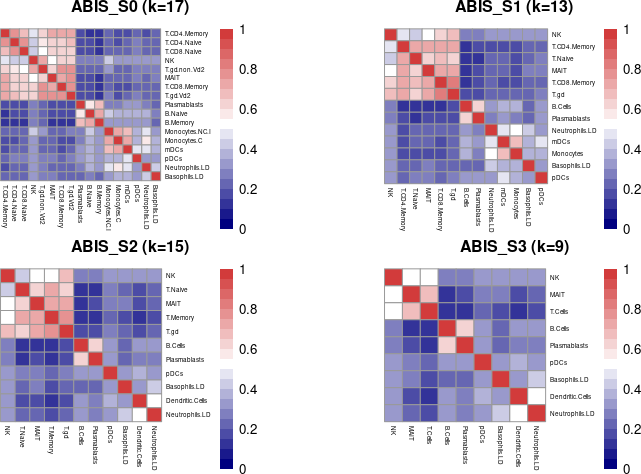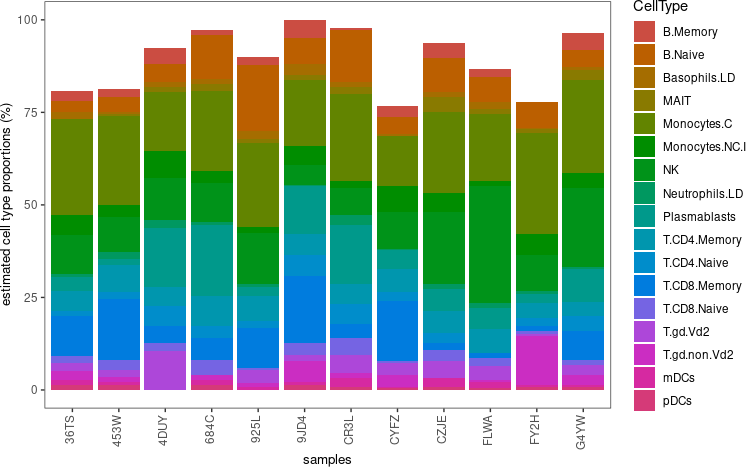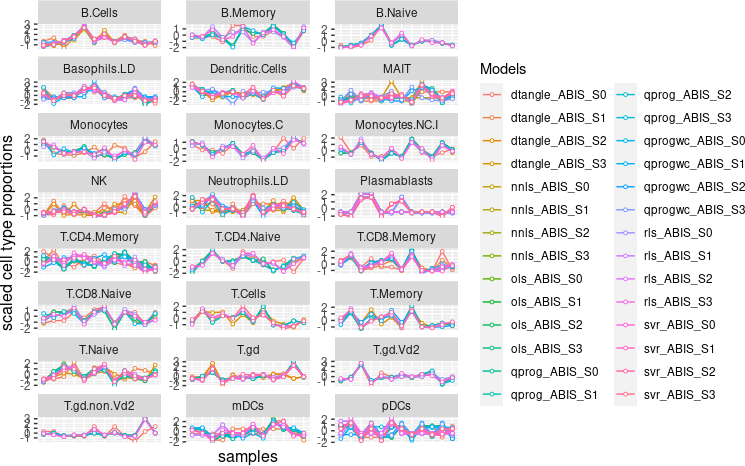# 1 Introduction

Bulk transcriptomic data is often generated from heterogeneous samples composed of multiple cell types, where measured values represent an averaged gene expression across all cell types. This heterogeneity is a major hurdle in the statistical analysis, as differences in cell type proportions may prevent or bias the detection of cell type-specific transcriptional programs.

In silico deconvolution of bulk gene expression data allows to infer cell type composition of heterogeneous biological samples. Deconvolution methods are typically used to estimate cell type fractions in bulk RNA-seq data from whole blood, peripheral blood mononuclear cells or other complex tissues in healthy and diseased patients (Abbas et al. 2009; Shen-Orr et al. 2010). Estimated cell type proportions can then be used in subsequent analysis to correct for cell-type heterogeneity making in silico deconvolution an attractive alternative to the physical isolation of individual cell types or single cell RNA-seq.

Several deconvolution methods have been published in recent years, many of which use cell type-specific gene expression references. In this vignette, we present granulator, an R package that provides a unified testing interface to rapidly run and benchmark multiple state-of-the-art deconvolution methods (Table 1).

Table 1: Deconvolution methods
List of deconvolution algorithms available in granulator.
ols stats::lsfit Ordinary least squares free (GPL-2)
nnls nnls::nnls Non-negative least squares free (GPL-2, GPL-3) reimplemented based on (Abbas et al. 2009)
qprogwc limSolve::lsei Quadratic programming with non-negativity and sum-to-one constraint free (GPL-2, GPL-3) reimplemented based on (Gong and Szustakowski 2013)
qprog limSolve::Solve Quadratic programming without constraints free (GPL-2, GPL-3)
rls MASS::rlm Re-weighted least squares free (GPL-2, GPL-3) reimplemented based on (Monaco et al. 2019)
svr e1071::svr Support vector regression free (GPL-2, GPL-3) reimplemented based on (Newman et al. 2015)
dtangle dtangle::dtangle Linear mixing model free (GPL-3) (Hunt et al. 2018)

Each deconvolution method takes as input bulk expression profiles of mixed tissue samples and a reference molecular profile of the individual cell types, which are used to estimate the abundance of cell types in each sample. In the following sections we show how to use granulator for the deconvolution of bulk RNA-seq data from peripheral blood mononuclear cells into the individual cellular components using public reference profiles (Table 2) and how to assess the quality of the obtained predictions.

Table 2: Reference profiles
List of reference profiles available in granulator.
Name Description Reference
sigMatrix_ABIS_S0 PBMCs reference profile (17 cell types) (Monaco et al. 2019)
sigMatrix_ABIS_S1 PBMCs reference profile (13 cell types)
sigMatrix_ABIS_S2 PBMCs reference profile (11 cell types)
sigMatrix_ABIS_S3 PBMCs reference profile (9 cell types)

# 2 Installation

granulator can be installed from Bioconductor using:

``````if (!requireNamespace("BiocManager", quietly = TRUE))
install.packages("BiocManager")
BiocManager::install("granulator")``````

The package can be loaded using:

``library(granulator)``

# 3 Data

The datasets used in this vignette comprises bulk RNA-seq gene expression data of peripheral blood mononuclear cells (PBMCs) from 12 healthy donors and bulk RNA-seq data of 29 isolated immune cell types from 4 healthy donors (Monaco et al. 2019), publicly available on the NCBI database under GEO accession number GSE107011. For convenience, a subset of the data is included in the package and can be loaded by using the function `load_ABIS()`:

``````# load datasets for deconvolution of PBMC RNA-seq data

## 3.1 Bulk PBMCs RNA-seq

A subset of the PBMCs bulk RNA-seq data, stored in `bulkRNAseq_ABIS`, consists of a gene (rows) by sample (columns) matrix with transcript-per-million (TPM) gene expression values:

``````# print TPM values in bulk RNA-seq
bulkRNAseq_ABIS[1:5, 1:5]``````
``````##              CYFZ      FY2H       FLWA      453W       684C
## S1PR3    4.275496 11.544026 10.4491331 13.192052  8.0657227
## RXFP2    2.038530  3.434462  5.4659900  2.877763  2.8150600
## ADAMTS5  0.010506  0.000000  0.1700436  0.000000  0.6866893
## CLEC6A   4.786615  9.357342  6.1288175  8.606988  4.4801144
## FXYD6   19.881627 29.860584 20.3102595 26.095918 22.7797553``````

## 3.2 Reference profiles

We use the reference profile from isolated cell types for 17 immune cell types. The PBMCs reference profile, stored in `sigMatrix_ABIS_S0`, consists of a gene (rows) by cell type (columns) matrix containing transcript-per-million (TPM) gene expression values normalized for total mRNA abundance:

``````# print TPM values in reference profile matrix
sigMatrix_ABIS_S0[1:5, 1:5]``````
``````##         Monocytes.C        NK T.CD8.Memory T.CD4.Naive T.CD8.Naive
## S1PR3     45.720735 0.2790023    0.1981103   0.3657506   0.1930285
## RXFP2     17.877398 0.0000000    0.0000000   0.0000000   0.0000000
## ADAMTS5    2.550237 0.0000000    0.0000000   0.0000000   0.0000000
## CLEC6A    33.695996 0.0000000    0.0000000   0.0000000   0.0000000
## FXYD6    114.167642 0.4707691    0.1832934   0.2908456   0.1365307``````

Additionally, we provide a set of reference profile matrices stored in `sigMatrix_ABIS_S1`, `sigMatrix_ABIS_S2`, and `sigMatrix_ABIS_S3`, which were derived at different levels of cell type resolution by summing over similar cell types.

## 3.3 Measured cell type proportions

Cell type proportions were measured by fluorescence-activated cell sorting (FACS) for 29 immune cell types (Monaco et al. 2019). Additional cell type proportions were computed by summing over cell types with highly similar transcriptional profiles. For instance `T.CD4.Naive` proportions consist of the weighted sum of the subtypes Th1, Th2, Th1/Th17, Tregs, Tfh, Naive CD4 T cells and Terminal Effector CD4 T cells.

The measured cell type proportions, stored in `groundTruth_ABIS`, consists of a sample (rows) by cell type (columns) matrix with proportions expressed in percent:

``````# print measured cell type proportions (percentages)
groundTruth_ABIS[1:5, 1:5]``````
``````##      Monocytes.C    NK T.CD8.Memory T.CD4.Naive T.CD8.Naive
## 453W        19.4  6.78       22.931       9.165       5.328
## 684C        19.6  8.45        7.078      10.051       8.411
## CR3L        14.0 10.80        3.597      10.871      11.532
## FLWA        19.6 19.50        4.530       6.084       3.815
## FY2H        26.8  2.60       12.008       8.098       5.279``````

# 4 Workflow

The granulator workflow consists of four steps:

1. Reference profiles: Reference profiles for deconvolution are usually generated by differential expression analysis on bulk RNA-seq generated from isolated cell types or cell-type clusters identified by single cell RNA-seq;

2. Deconvolution: Bulk RNA-seq data from heterogeneous samples is than deconvoluted using one or more reference profiles and deconvolution methods;

3. Benchmarking: Estimated cell type proportions are benchmarked against measured cell type proportions to assess deconvolution performance. Measured proportions are usually generated from fluorescence-activated cell sorting or single cell RNA-seq data;

4. Correlation: Benchmarked reference profiles can be used to deconvolve bulk RNA-seq data where individual cell types abundances are unknown. The deconvoluted cell type proportions can be correlated with each other in order to assess the degree of similarity in predictions across methods.

## 4.1 Comparing multiple reference profiles

The performance of cell type deconvolution strongly depends on the choice and quality of the reference profile, and in particular on the degree of similarity between cell-type specific expression profiles (Vallania et al. 2018; Avila Cobos et al. 2020). It is therefore recommended to test multiple reference profile matrices generated at different cell type resolutions (Newman et al. 2019; Monaco et al. 2019). Here we use the published `sigMatrix_ABIS_S0` reference profile, and additional signatures generated by collapsing highly similar cell types into single categories (`sigMatrix_ABIS_S1`, `sigMatrix_ABIS_S2`, `sigMatrix_ABIS_S3`).

``````# create list if multiple signature matrices to test simultaneously
sigList = list(
ABIS_S0 = sigMatrix_ABIS_S0,
ABIS_S1 = sigMatrix_ABIS_S1,
ABIS_S2 = sigMatrix_ABIS_S2,
ABIS_S3 = sigMatrix_ABIS_S3)``````

We plot the cell-type similarity matrix of all reference profiles by computing their Kendall Rank Correlation Coefficient with `plot_similarity()`, highlighting clusters of transcriptionally related cell types:

``````# plot signature matrix similarity matrices
plot_similarity(sigMatrix=sigList)``````A useful metric to evaluate the quality of reference profile matrices is to compute the Condition Number `k`, which measures how sensitive the deconvolution is to variability in the input data. Generally, a matrix with low condition number (`k` close to 1) is well-conditioned, as it leads to a stable solution.

## 4.2 Deconvolution of bulk RNA-seq data

Once suitable reference profiles have been generated, we use `deconvolute()` to estimate cell type proportions from the tissue bulk RNA-seq dataset. The function takes a matrix dataset to be deconvoluted, a matrix or a list of reference profile matrices, and a vector of deconvolution methods. All data matrices need to be normalized to TPM from raw counts with the function `get_TPM()`. By default, `deconvolute()` sequentially runs all methods available. Optionally, we can provide a selected list of methods and the number of available processing cores to minimize computation time. Every reference profile matrix is tested in combination with every selected method.

``````# deconvolute input data using all available methods by default
decon <- deconvolute(m = bulkRNAseq_ABIS, sigMatrix = sigList)``````

For each reference profile and method combination, the function returns the estimated cell type `coefficients` and `proportions` (in percentage). Although there may be slightly negative proportions, significant negative values means that deconvolution mehtods fails to converge on a biological meaningful solution, and the reference profile matrix should be further refined.

We can look at the cell type proportions computed by the support vector regression model (`svr`) using the `sigMatrix_ABIS_S0` reference profile:

``````# print cell type proportions for svr model on ABIS_S0 reference profile
decon\$proportions\$svr_ABIS_S0[1:5, 1:5]``````
``````##      B.Memory B.Naive Basophils.LD MAIT Monocytes.C
## 36TS     2.73    3.18         1.82 0.00       25.91
## 453W     2.27    4.09         0.45 0.45       24.09
## 4DUY     4.09    5.00         1.36 1.36       15.91
## 684C     1.36   11.82         1.36 1.82       21.82
## 925L     2.27   17.73         2.27 0.91       22.73``````

We can plot the estimated cell type proportions with the function `plot_proportions()`. Notice that while the sum of cell types proportions cannot exceed 100%, for some methods part of the bulk RNA-seq signal remains unassigned.

``````# plot cell type proportions for svr model on ABIS_S0 reference profile
plot_proportions(deconvoluted = decon, method = 'svr', signature = 'ABIS_S0')``````To plot all estimated cell type proportions we use the function `plot_deconvolute()`, which allows to compare results across deconvolution methods and cell types. The option `scale` indicates whether cell type proportions should be transformed into standard scores. Scaling is useful to directly compare deconvolution output, as the absolute percentages may vary considerably across methods.

``````# plot cell type proportions
plot_deconvolute(deconvoluted = decon, scale = TRUE, labels = FALSE)``````## 4.3 Benchmarking of deconvolution methods

The third step in the workflow is dedicated to assessing the performance of deconvolution by taking advantage of available known cell types abundances. We benchmark deconvolution methods by regressing the estimates against the measured cell type proportions with the function `benchmark()`:

``````# benchmark methods by correlating estimated to measured cell type proportions
bench <- benchmark(deconvoluted = decon, ground_truth = groundTruth_ABIS)``````

Summary statistics of the regression models by method, signature, and cell type can be displayed as follows:

``````# print metrics
``````##   signature  method       celltype    pcc    ccc adj.r2   rmse
## 1   ABIS_S0 dtangle       B.Memory 0.6256 0.3488  0.330 0.0083
## 2   ABIS_S0 dtangle        B.Naive 0.9620 0.9265  0.920 0.0062
## 3   ABIS_S0 dtangle   Basophils.LD 0.9095 0.7520  0.810 0.0031
## 4   ABIS_S0 dtangle           MAIT 0.8004 0.6796  0.600 0.0075
## 5   ABIS_S0 dtangle    Monocytes.C 0.4210 0.3101  0.095 0.0320
## 6   ABIS_S0 dtangle Monocytes.NC.I 0.8373 0.8280  0.670 0.0085``````

We can also print the average metrics by regression method and signature as follows:

``````# print metrics
``````##   signature method mean_pcc mean_ccc mean_adj.r2 mean_rmse
## 1   ABIS_S2   nnls   0.8299   0.2093      0.6645    0.0118
## 2   ABIS_S2    ols   0.8298   0.2121      0.6636    0.0129
## 3   ABIS_S2  qprog   0.8298   0.2121      0.6636    0.0129
## 4   ABIS_S2    svr   0.8292   0.4746      0.6655    0.0161
## 5   ABIS_S3    svr   0.8045   0.4305      0.6211    0.0180
## 6   ABIS_S2    rls   0.7922   0.2129      0.6155    0.0124``````

Evaluation metrics include the Pearson Correlation Coefficient (`pcc`), the Concordance Correlation Coefficient (`ccc`), the Coefficient of Determination (`adj.r2`), and the Root Mean Square Error (`rmse`). When a cell type cannot be deconvoluted, it’s proportions are returned as `NA`, which causes the corresponding metric coefficients to be missing as well.

While `pcc` measures the linear correlation between relative changes in proportions across all samples, `ccc` measures the linear correlation between true and estimated proportions by taking the mean absolute percentages into account. Both `pcc` and `ccc` metrics can range between 1 and -1: a value of 1 represents a total positive correlation, 0 no correlation, and −1 a total negative correlation. `adj.r2` represents the square of `pcc` adjusted for the number of predictors in the model and takes values between 0 and 1. Conversely the `rmse` measures the quadratic mean of the differences between predicted values and observed values. A value of 0.05 represent a difference of 5%.

The linear regression of estimated versus measured cell type proportions can be visualized using `plot_regress()` on the `benchmark()` results. Here, we analyze the performance of the support vector regression model (`svr`) across the deconvoluted cell types using the `sigMatrix_ABIS_S0` reference profile:

``````# plot regression for svr model on ABIS_S0 reference profile
plot_regress(benchmarked = bench, method = 'svr', signature = 'ABIS_S0')``````